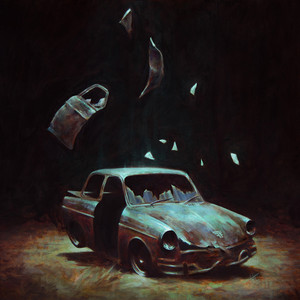Clair de Luneby Flight Facilities (Feat. Christine Hoberg) Lyrics

Released
Oct 18, 2013
Genre
Dance

Clair de Lune Lyrics

Song Meaning
Verse 1
If I would know you, would you know me,
If I would know you, would you know me,
If I would know you, would you know me,
If I would know you, would you know me
Verse 2
Don't go, tell me that the lights won't change,
Tell me that you'll feel the same, and we'll stay here forever,
Don't go, tell me that the lights won't change,
Tell me that it'll stay the same,
Where we go, where we,
Where we go, where we go,
Where we go, where we go, where we go.
Verse 3
[Repeat x4]
Where we go, where we,
Where we go, where we,
Where we go, where we,
Where we go, where we go
Verse 4
Don't go, tell me that the lights won't change,
Tell me that you'll feel the same, and we'll stay here forever,
Don't go, tell me that the lights won't change,
Tell me that it'll stay the same,
Where we go, where we,
Where we go, where we go,
Where we go, where we go, where we go.
Verse 5
If I would know you, would you know me,
If I would know you, would you know me,
If I would know you, would you know me,
If I would know you, would you know me
Verse 6
Don't go, tell me that the lights won't change,
Tell me that we'll stay the same,
Where we go, where we,
Where we go, where we go,
Where we go, where we go, where we go.

Lyrics Licensed & Provided by LyricFindNo Thoughts or Song Meanings Yet

Be the first to leave a thought.

Thoughts

1

1

1

1

1

1

1

1

1

Views

1039

963

179

165

86